Standard Algorithm with Decimals (All 4 Operations) Video - Grades 6-8
1%
It was processed successfully!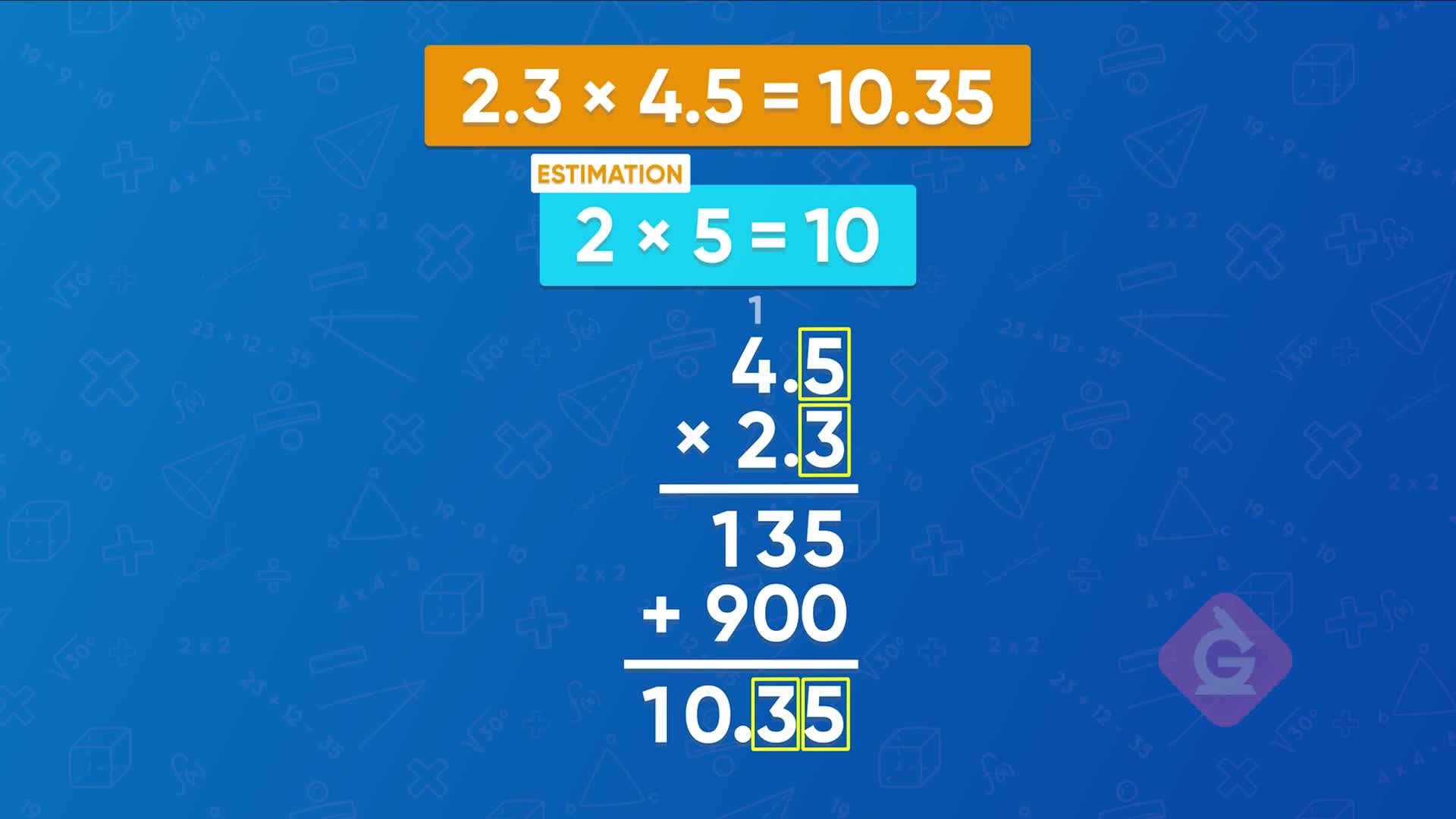WHAT IS THE STANDARD ALGORITHM WITH DECIMALS?

Adding, subtracting, multiplying, and dividing with decimals is not very different from operations with whole numbers. If we follow the standard algorithms, pay attention to place value, and think about where the decimal point should end up, we can find the answers.

To better understand the standard algorithm with decimals…

WHAT IS THE STANDARD ALGORITHM WITH DECIMALS?. Adding, subtracting, multiplying, and dividing with decimals is not very different from operations with whole numbers. If we follow the standard algorithms, pay attention to place value, and think about where the decimal point should end up, we can find the answers. To better understand the standard algorithm with decimals…

## LET’S BREAK IT DOWN!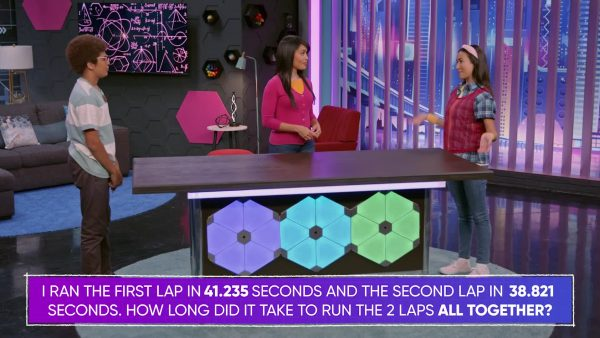Emily is on the track team. She runs the first lap in 41.235 seconds, and the second lap in 38.821 seconds. Add to find out how long it took her to run both laps together. Line up the two numbers so that the place values are aligned, and then add one place value at a time, starting with the digits that have the least value. When a sum is 10 or greater, regroup a 1 to the next place value. 41.235 + 38.821 = 80.056 seconds. Now you try: 1.724 + 2.193 = ?

Adding decimals Emily is on the track team. She runs the first lap in 41.235 seconds, and the second lap in 38.821 seconds. Add to find out how long it took her to run both laps together. Line up the two numbers so that the place values are aligned, and then add one place value at a time, starting with the digits that have the least value. When a sum is 10 or greater, regroup a 1 to the next place value. 41.235 + 38.821 = 80.056 seconds. Now you try: 1.724 + 2.193 = ?

### When the numbers have different place values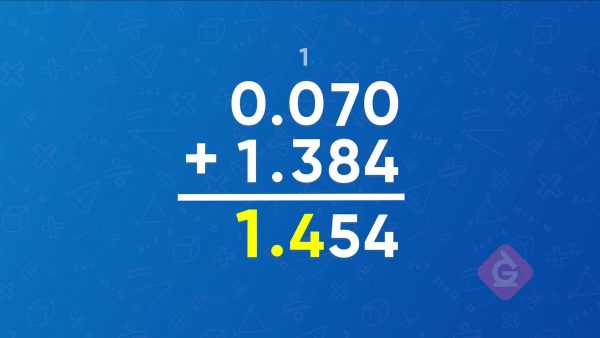To add 0.07 + 1.384, we still have to line the numbers up by place value. 0.07 doesn’t have a digit in the thousandths place, so it is easiest to start by aligning the decimal points. Now all our place values are aligned, and we add a 0 at the end of 0.07 to make 0.070. That does not change the value but makes the numbers easier to add. 0.07 + 1.384 = 1.454. Now you try: 2.04 + 10.123 = ?

When the numbers have different place values To add 0.07 + 1.384, we still have to line the numbers up by place value. 0.07 doesn’t have a digit in the thousandths place, so it is easiest to start by aligning the decimal points. Now all our place values are aligned, and we add a 0 at the end of 0.07 to make 0.070. That does not change the value but makes the numbers easier to add. 0.07 + 1.384 = 1.454. Now you try: 2.04 + 10.123 = ?

### Subtracting decimals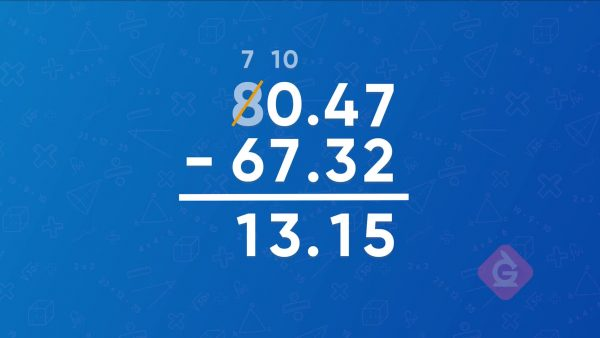You had \$80.47. You spent \$67.32. Subtract to find how much money you have left. Start by lining up the numbers by place value, with the greater number on top. Then subtract the digits, starting with the place value on the right. When we get to the ones place, there is 0 – 7. Since there are not enough ones to subtract 7 ones, regroup 1 ten as 10 ones. Now we have 10 – 7 = 3. The tens place is now 7 instead of 8. Finally, subtract the tens. The final answer is 13.15, which means you have \$13.15 left. Now you try: 21.90 – 15.12 = ?

Subtracting decimals You had \$80.47. You spent \$67.32. Subtract to find how much money you have left. Start by lining up the numbers by place value, with the greater number on top. Then subtract the digits, starting with the place value on the right. When we get to the ones place, there is 0 – 7. Since there are not enough ones to subtract 7 ones, regroup 1 ten as 10 ones. Now we have 10 – 7 = 3. The tens place is now 7 instead of 8. Finally, subtract the tens. The final answer is 13.15, which means you have \$13.15 left. Now you try: 21.90 – 15.12 = ?

### Multiplying decimalsYou feed your dog 2.3 cups of food a day, and you need to bring enough food for a 4.5-day trip. Start by multiplying the digits, ignoring the decimal points, using the standard algorithm. 23 × 45 = 1,035. To decide where to put the decimal point, find the total number of decimal places in the original numbers: 2. The decimal point goes two places from the right. The answer is 10.35. Now you try: 1.7 × 1.5 = ?

Multiplying decimals You feed your dog 2.3 cups of food a day, and you need to bring enough food for a 4.5-day trip. Start by multiplying the digits, ignoring the decimal points, using the standard algorithm. 23 × 45 = 1,035. To decide where to put the decimal point, find the total number of decimal places in the original numbers: 2. The decimal point goes two places from the right. The answer is 10.35. Now you try: 1.7 × 1.5 = ?

### Dividing decimals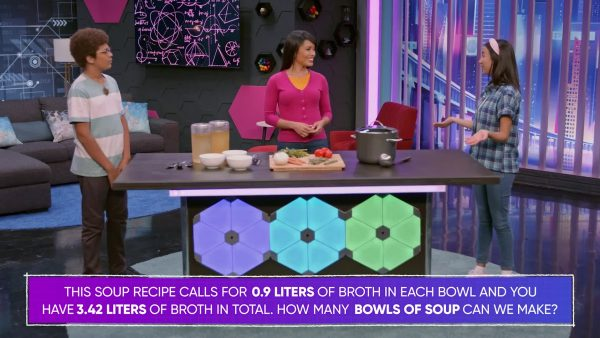You want to divide 3.42 liters of soup into bowls that each hold 0.9 liter. Divide 3.42 by 0.9 to find the number of bowls you can fill. Change the problem into an equivalent problem with a whole number divisor. Multiply the divisor and dividend both by 10 to get 34.2 ÷ 9. Now divide using the standard algorithm for division. 34.2 ÷ 9 = 3.8. You can fill 3 bowls and 0.8 of a fourth bowl. Now you try: 2.24 ÷ 0.4 = ?

Dividing decimals You want to divide 3.42 liters of soup into bowls that each hold 0.9 liter. Divide 3.42 by 0.9 to find the number of bowls you can fill. Change the problem into an equivalent problem with a whole number divisor. Multiply the divisor and dividend both by 10 to get 34.2 ÷ 9. Now divide using the standard algorithm for division. 34.2 ÷ 9 = 3.8. You can fill 3 bowls and 0.8 of a fourth bowl. Now you try: 2.24 ÷ 0.4 = ?

## STANDARD ALGORITHM WITH DECIMALS (ALL 4 OPERATIONS) VOCABULARY

Place value
The value of a digit based on its position in a number.
Decimal point
The dot that separates whole numbers from parts of a whole.
Algorithm
Sum
The result when numbers are added together.
Dividend
The number that we divide in a division expression.
Divisor
The number that we divide by in a division expression.
Split into equal parts.

## STANDARD ALGORITHM WITH DECIMALS (ALL 4 OPERATIONS) DISCUSSION QUESTIONS

### If you want to add 3.51 + 5.21, how should you line them up?

I should line them up by place value. The decimal points should also be lined up exactly.

### What if you want to add numbers that have a different number of decimal places, like 2.5 + 4.123?

I still want to line the numbers up by place value! If there is no value in a place, I can write a zero in that place. So I add 2.500 + 4.123.

### If you want to multiply 4.39 × 5.1, should you line the numbers up by place value?

Not this time! I should line them up to the right so that the 9 is above the 1. I ignore the decimal point until I am done calculating.

### How do you know where to put the decimal point at the end of multiplying?

I look at how many decimal places each factor has. Add them together, and that is how many decimal places the product should have. I can also find out by rounding and estimating the answer.

### What should you do before starting long division on numbers that have decimal points?

Multiply the divisor by a power of 10 until it is a whole number. Then multiply the dividend by the same power of 10. Now I can start the long division algorithm.
X

## Success

We’ve sent you an email with instructions how to reset your password.
Ok

## Choose Your Free Trial Period### 7 DaysContinue to Lessons### 90 DaysGet 90 days free by inviting other teachers to try it too.

Share with Teachers

## Get 90 Days Free

### By inviting 4 other teachers to try it too.

4 required

Skip, I will use a 7 days free trial

## Thank You!

Enjoy your free 90 days trial